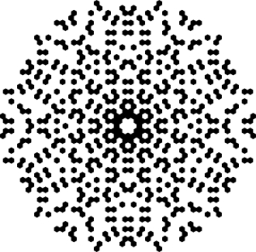# AND-NOT-AND

If P is the set of multiples of 2, Q is the set of multiples of 3, and R is the set of multiples of 7, the following integers will be in P and Q but not in R:

A=−54
B=−50
C=42
D=100
E=252

Result

x = (Correct answer is: ABD)### Step-by-step explanation:Did you find an error or inaccuracy? Feel free to write us. Thank you!

Tips for related online calculators
Do you want to perform natural numbers division - find the quotient and remainder?